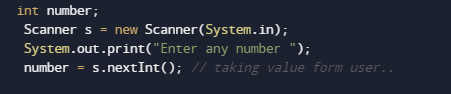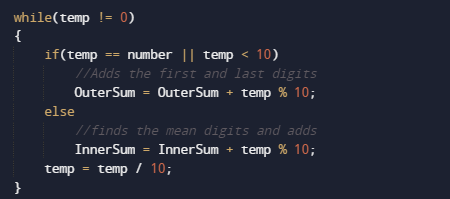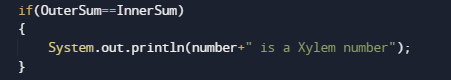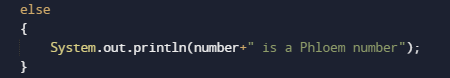# Program to check Xylem and Phloem Number In Java

In this tutorial you will learn about the Program to check Xylem and Phloem Number In Java and its application with practical example.

In this tutorial, we will learn to create a Java Program to check Xylem and Phloem Number In Java using Java programming.

## Prerequisites

Before starting with this tutorial we assume that you are best aware of the following Java programming topics:

• Java Operators.
• Basic Input and Output function in Java.
• Class and Object in Java.
• Basic Java programming.
• If-else statements in Java.
• For loop in Java.
• User define functions.

## Xylem and Phloem Number

A number will be called a xylem number if sum of its outermost number i.e (first and last) digits is equivalent to the sum of internal digit (except first and last) digits. If the sum of Outer digits is not equal to the sum of remaining internal digits, then the number is called phloem number.

Example:
12225: 1+5 = 6 and  2+2+2= 6  Xylem number
12235 : 1+5 = 6 and  2+2+3= 7 Phloem number.

## Program to check Xylem and Phloem Number In Java

In this program we will create a program to check weather the number is Xylem or Phloem. We would first declared and initialized the required variables. Next, we would prompt user to input the two values . Later we will find that number is Xylem or Phloem Number In Java.

## Xylem Number## Phloem NumberIn the above program, we have first declared and initialized a set variables required in the program.

• number = number to hold given value.
• temp =it will hold temporary the original number
• OuterSum= this will hold sum of outer values.
• InnerSum= It will sum of all internal values.

In the next statement user will prompted to enter a number which will be assigned to variable ‘number’. As shown in image below.## Logic to find Xylem and Phloem Number

• taking and initialize a number to variable “number”.
• Then find the outermost digits of number from both side i.e (first and last).
• Add the outer digits and store them in OuterSum.
• And afterwords Find the sum of  internal Digits of number (all number except first and last) in InnerSum.
• Compare both the sums(OuterSum,InnerSum).
• If they are equal, the number is a Xylem Number
• Else, the number is a Phloem number.At last we will display the result if outer and inner sum are equal then the number is Xylem Number.if not , the number is a Phloem number.In this tutorial we have learn about the Program to check Xylem and Phloem Number In Java and its application with practical example. I hope you will like this tutorial.